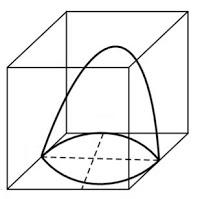# A hemispherical depression is cut out from one face of a cubical wooden block such that the diameter l of the hemisphere is equal to the edge of the cube. Determine the surface area of the remaining solid.The diameter of hemisphere = Edge of the cube = l

So, the radius of hemisphere = l/2

∴ The total surface area of solid = surface area of cube + CSA of hemisphere – Area of base of hemisphere

=6*side*side- πr2+2πr2

=6*l*l- πr2

=6l2-πr2

=6l– π(l/2)2

=6l2 – πl2/4

=(24l2+ πl2)/4

=l2(24+π)/4